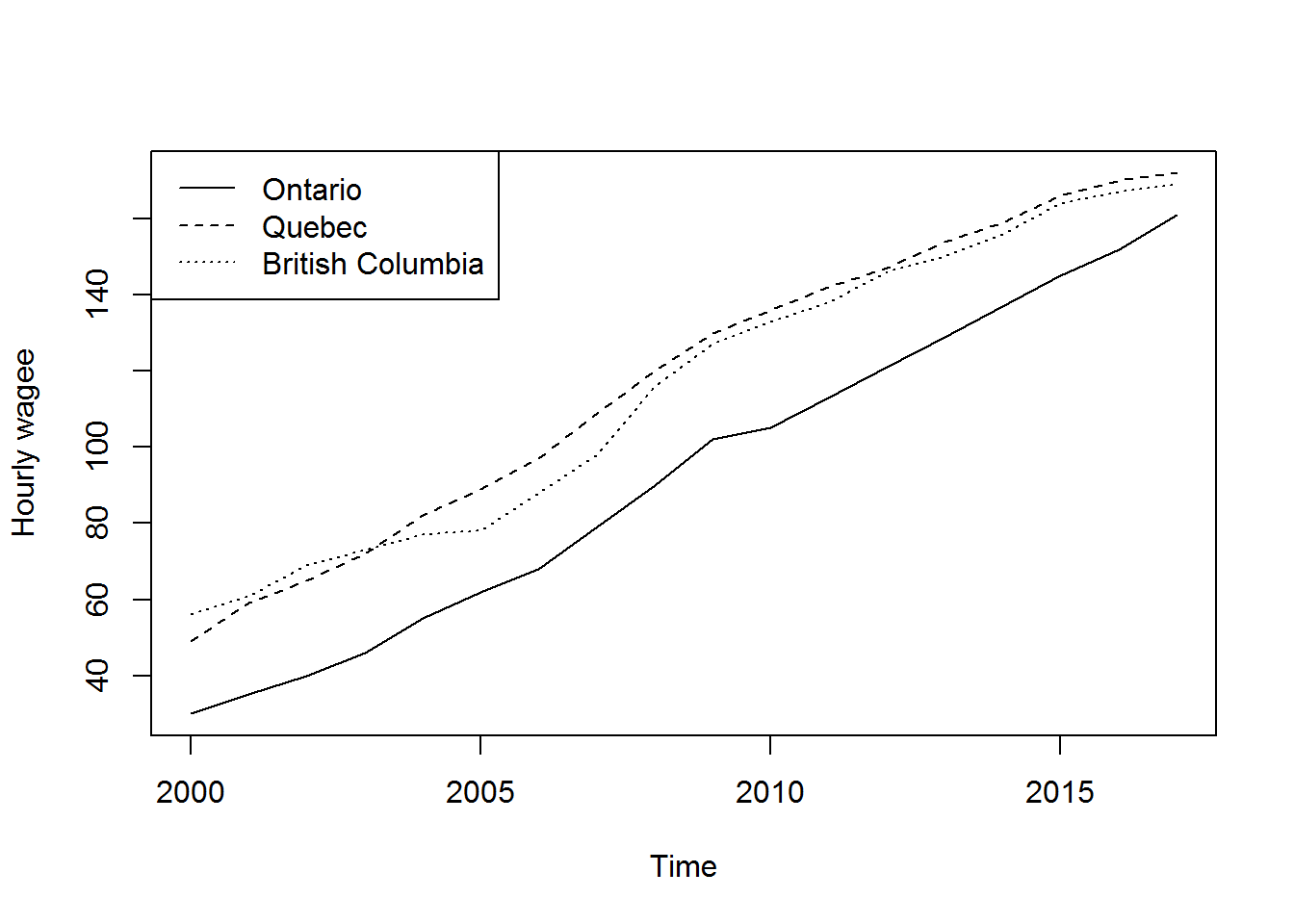# 1 - Basic Statistics.

## 1.1 - Crosstabs, distribution, marginal distribution.

#Read data file
LFSCanada$hrlyearn=LFSCanada$hrlyearn/100

### The function table() to create frequencies

It is possible to generate frequency tables using the table( ) function :

• The following table display the population by gender for each province.
#Average wage by age group
table1<-table(LFSCanada$prov,LFSCanada$sex)
table1
##
##                        Female  Male
##   Alberta                5476  5359
##   British Columbia       6228  5928
##   Manitoba               4614  4496
##   New Brunswick          2682  2511
##   Newfoundland           1972  1772
##   Nova Scotia            2871  2594
##   Ontario               14234 13297
##   Prince Edward Island   1446  1331
##   Québec                 8931  8577
##   Saskatchewan           3891  3680

### The function pro.table() to create tables of proportions.

• The option 1 will display row percentages: The sum of each row will be 1. In each province, we will have the proportion of Male and Female.
# Distribution of workers by gender for each province
tablepro1<-prop.table(table1,1)
round(tablepro1,digits = 2)
##
##                        Female Male
##   Alberta                0.51 0.49
##   British Columbia       0.51 0.49
##   Manitoba               0.51 0.49
##   New Brunswick          0.52 0.48
##   Newfoundland           0.53 0.47
##   Nova Scotia            0.53 0.47
##   Ontario                0.52 0.48
##   Prince Edward Island   0.52 0.48
##   Québec                 0.51 0.49
##   Saskatchewan           0.51 0.49

## 1.2 - Statistics : Mean, median, min, max.

### The function tapply() to create table of statistics

tapply() computes a measure (mean, median, min, max, etc..) or a function for each factor variable in a vector. The following example calculate the sample mean wage for each age group in Canada. We applied the special function tapply():

• x is a vector of the continuous variable : Individual wage.
• INDEX : list of factors : Age group.
• FUN : Is a function of statistics we want to display : Here we are looking mean. But it is possible also other statistics like median, percentiles.
#Average wage by age group
table3 <- tapply(LFSCanada$hrlyearn,INDEX = LFSCanada$age_12,FUN=mean,na.rm =TRUE)
round(table3,digits = 2)
## 15-19 20-24 25-29 30-34 35-39 40-44 45-49 50-54 55-59 60-64 65-69   70+
## 13.44 17.36 24.18 27.60 29.08 30.01 29.94 29.29 28.29 26.61 25.29 22.57

#### The function barplot to represent the previous table.

• Create the table you want to plot.
• Plot the table.

barplot command has many options : look at the help.

#Average wage by age group
barplot(table3,las=2,ylab = "Hourly wage",xlab = "Age group")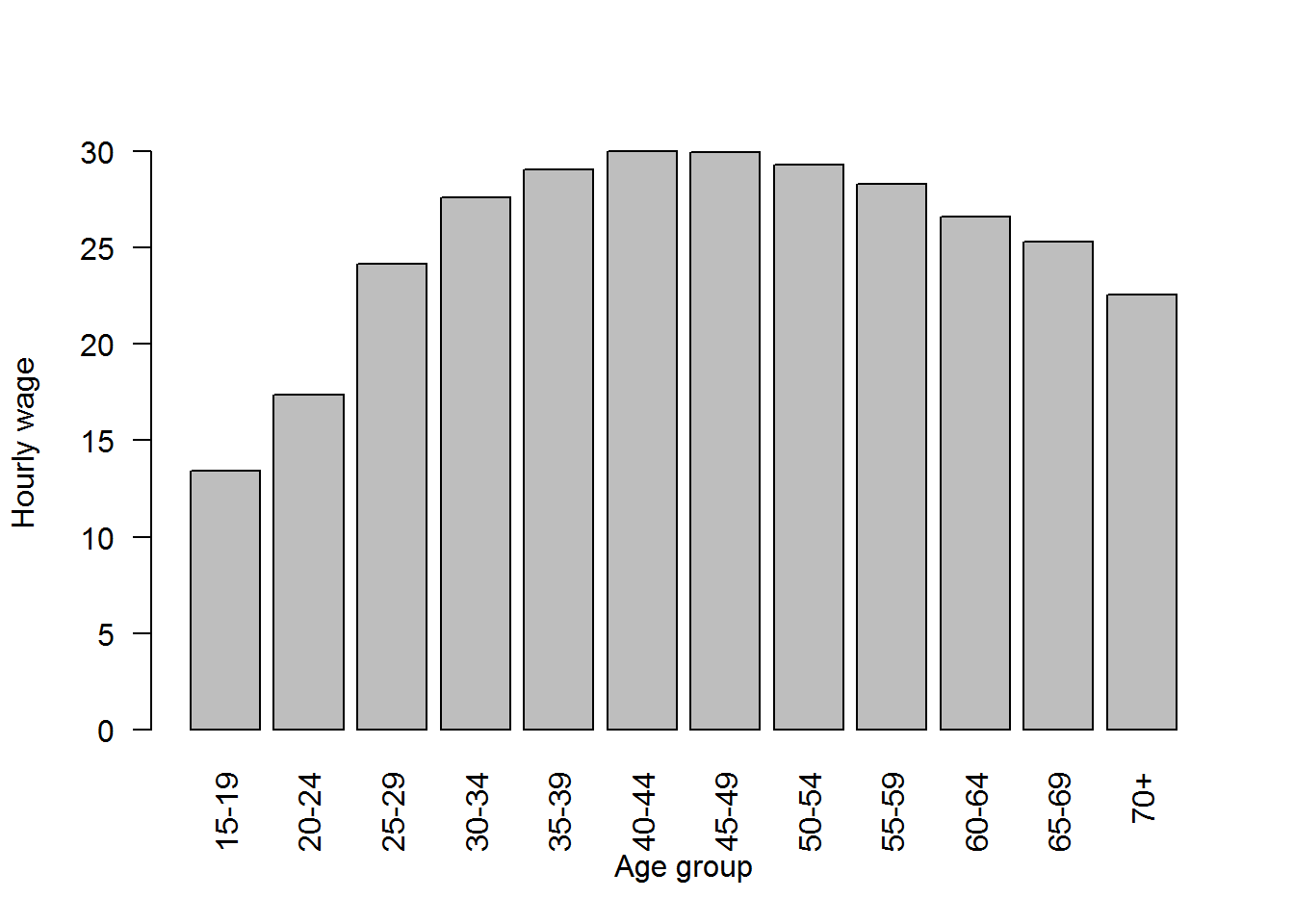#### Represent cross-tablebarplot with two variables

• Create the table you want to plot.
• Plot the table.

barplot command has many options : look at the help.

Table

#Average wage by age group
table4= tapply(LFSCanada$hrlyearn,INDEX=list(LFSCanada$sex,LFSCanada$age_12),FUN=mean,na.rm =TRUE) round(t(table4),digits = 2) ## Female Male ## 15-19 13.08 13.80 ## 20-24 16.59 18.10 ## 25-29 22.97 25.31 ## 30-34 25.87 29.23 ## 35-39 27.01 31.06 ## 40-44 27.73 32.27 ## 45-49 27.46 32.40 ## 50-54 26.77 32.02 ## 55-59 25.50 31.11 ## 60-64 23.91 29.03 ## 65-69 23.39 26.78 ## 70+ 20.53 24.09 Barplot #Average wage by age group barplot(table4,las=2,beside = TRUE) legend("topleft",legend = rownames(table4) , pch =c(15,15), col=c("gray10","gray70"))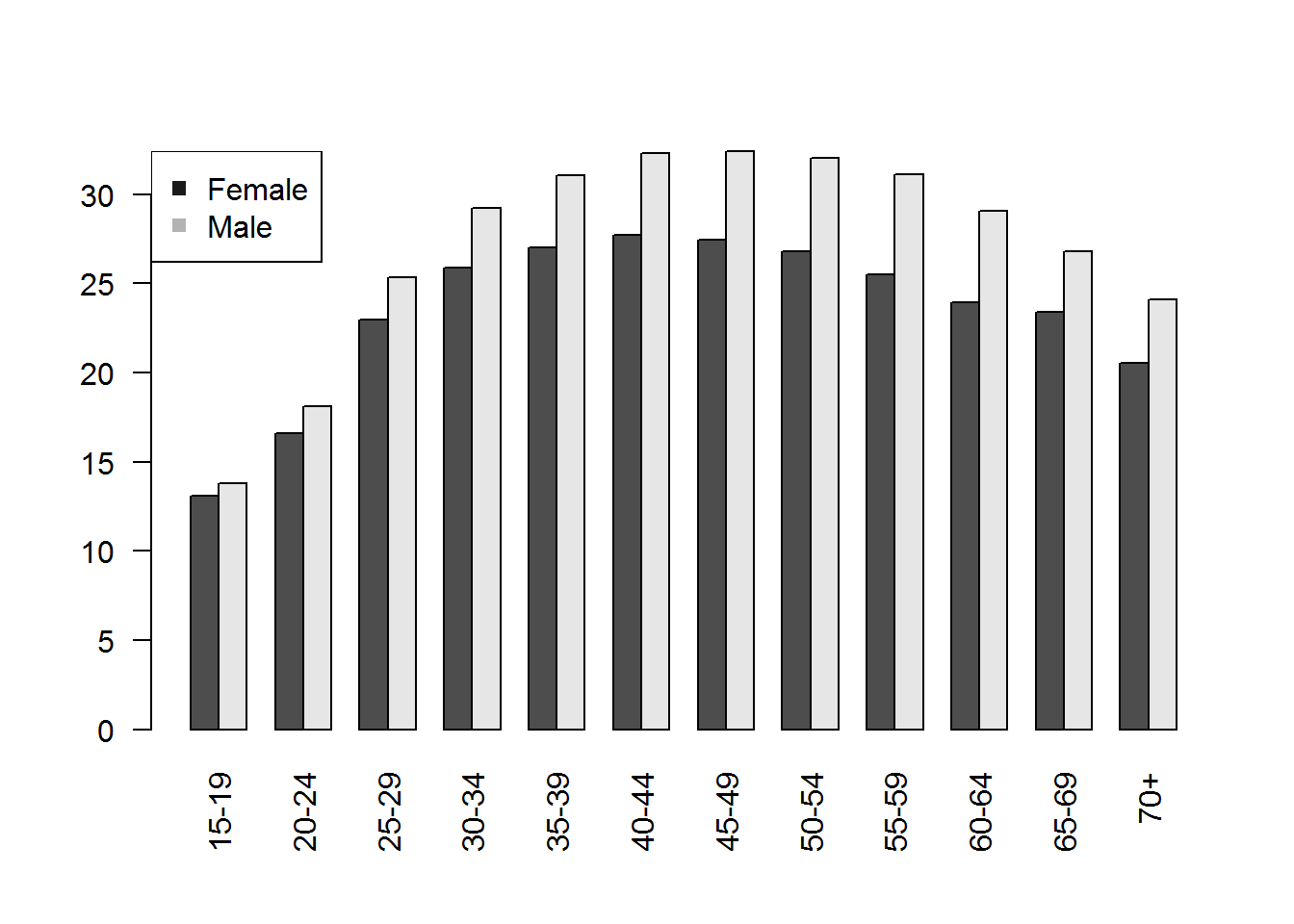# 2- Plotting Graphs. One of the most frequently used plotting functions in R is the plot() function. This is a generic function: the type of plot produced is dependent on the type or class of the first argument. ## Two continuous variables. If x and y are vectors, plot(x, y) produces a scatterplot of y against x : x=seq(0,20,0.5) e=rnorm(length(x),0,2) y=2+0.6*x+1.4*e plot(x,y)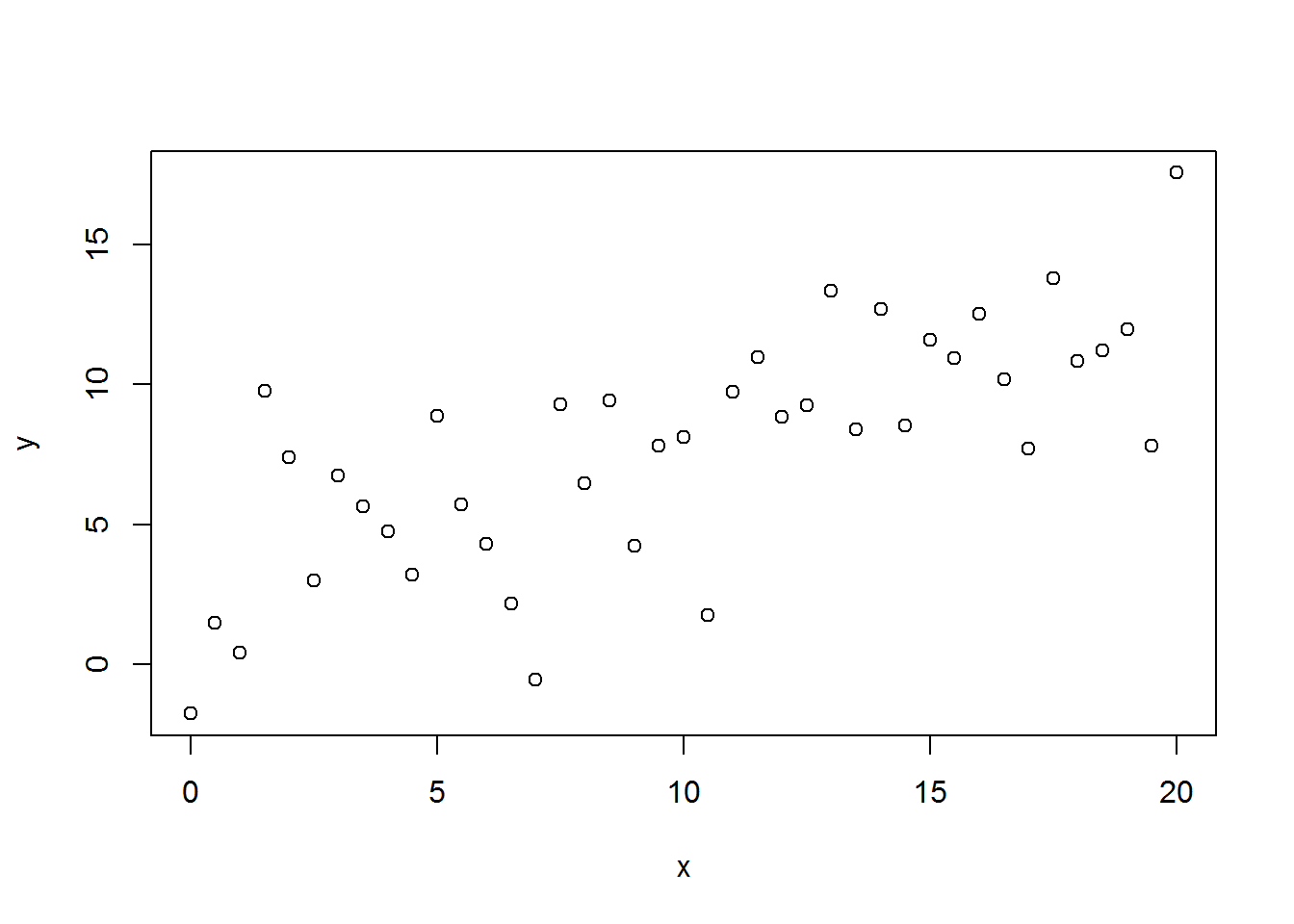Select individuals living in Quebec province #Read data file setwd("C:/Users/MamadouYaya/Dropbox/Fall2019/TA_Fall2019/Data") TSCanada <- read.csv2("TSCanada.txt", sep="") TSQuebec <-subset(TSCanada,prov=='Quebec') Plotting number of employed against wage during the period 2000-2017 for Quebec province : plot(TSQuebec$Employed,TSQuebec$hrlyearn,pch=24)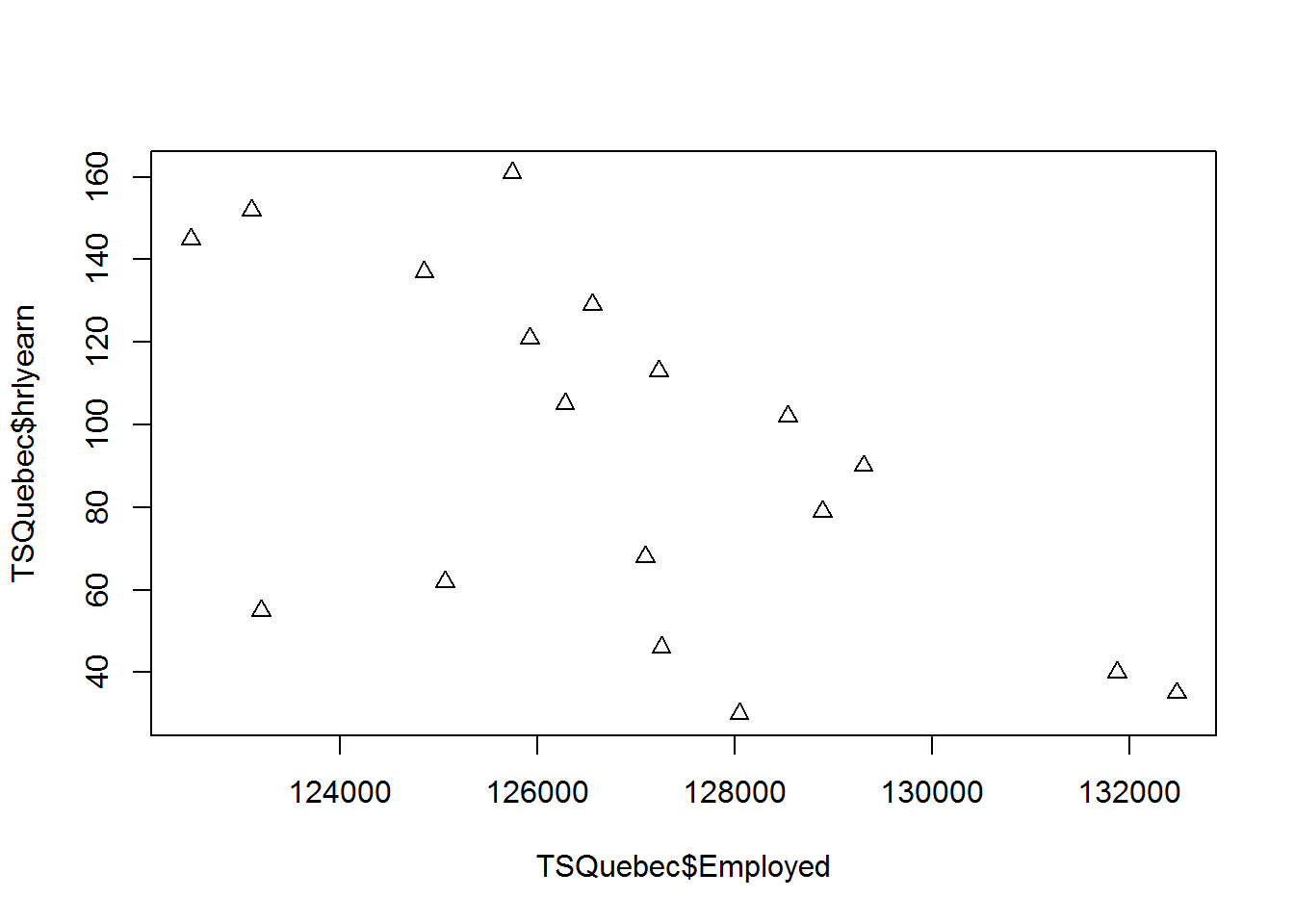Time-series : Trend of employment in Quebec from 2000 to 2017 plot(TSQuebec$year,TSQuebec$Employed, type = "l")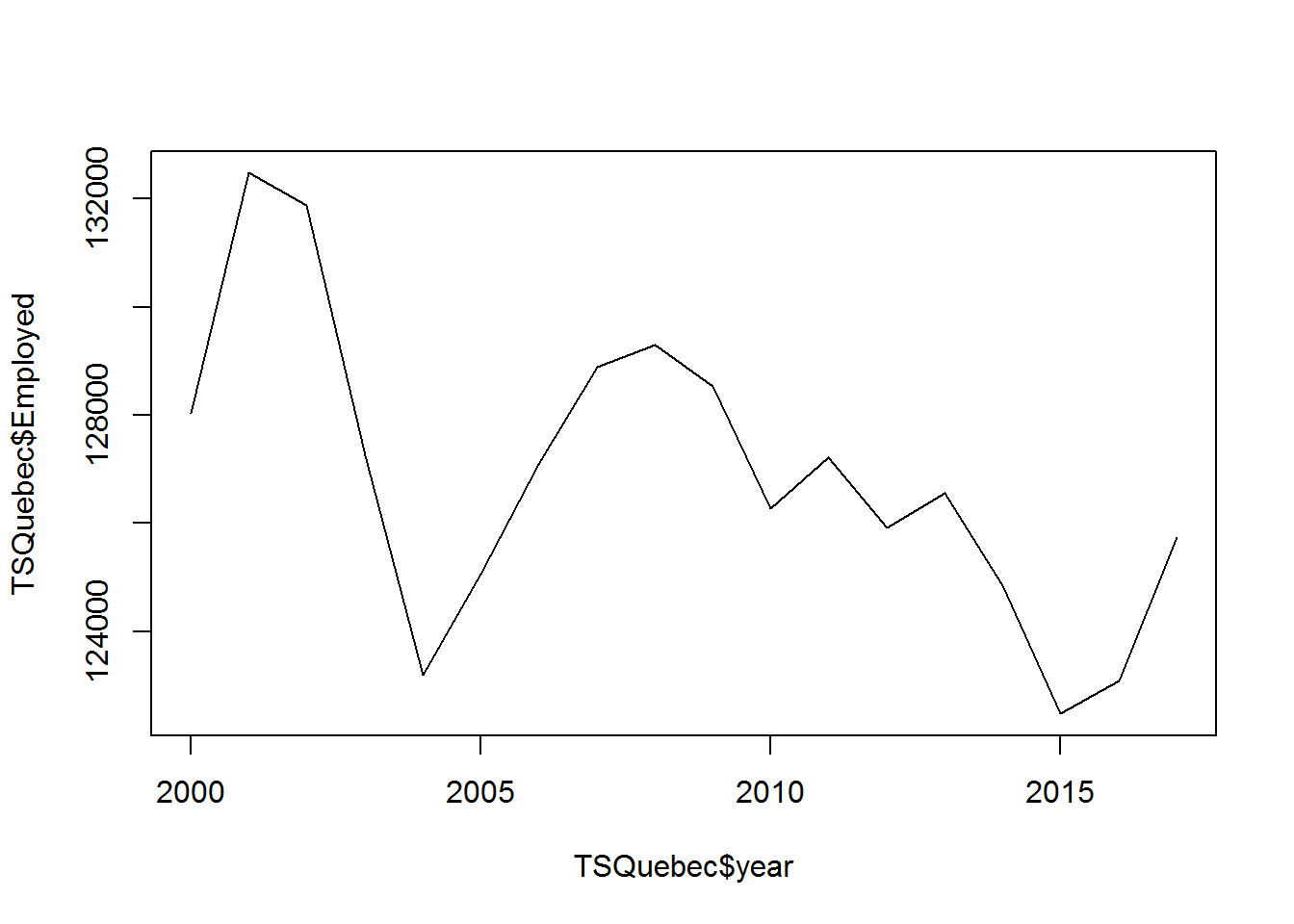Time-series : Trend (colored) of employment in Quebec from 2000 to 2017 plot(TSQuebec$year,TSQuebec$Employed, type = "l",col="blue",xlab="years", ylab="Number of employed")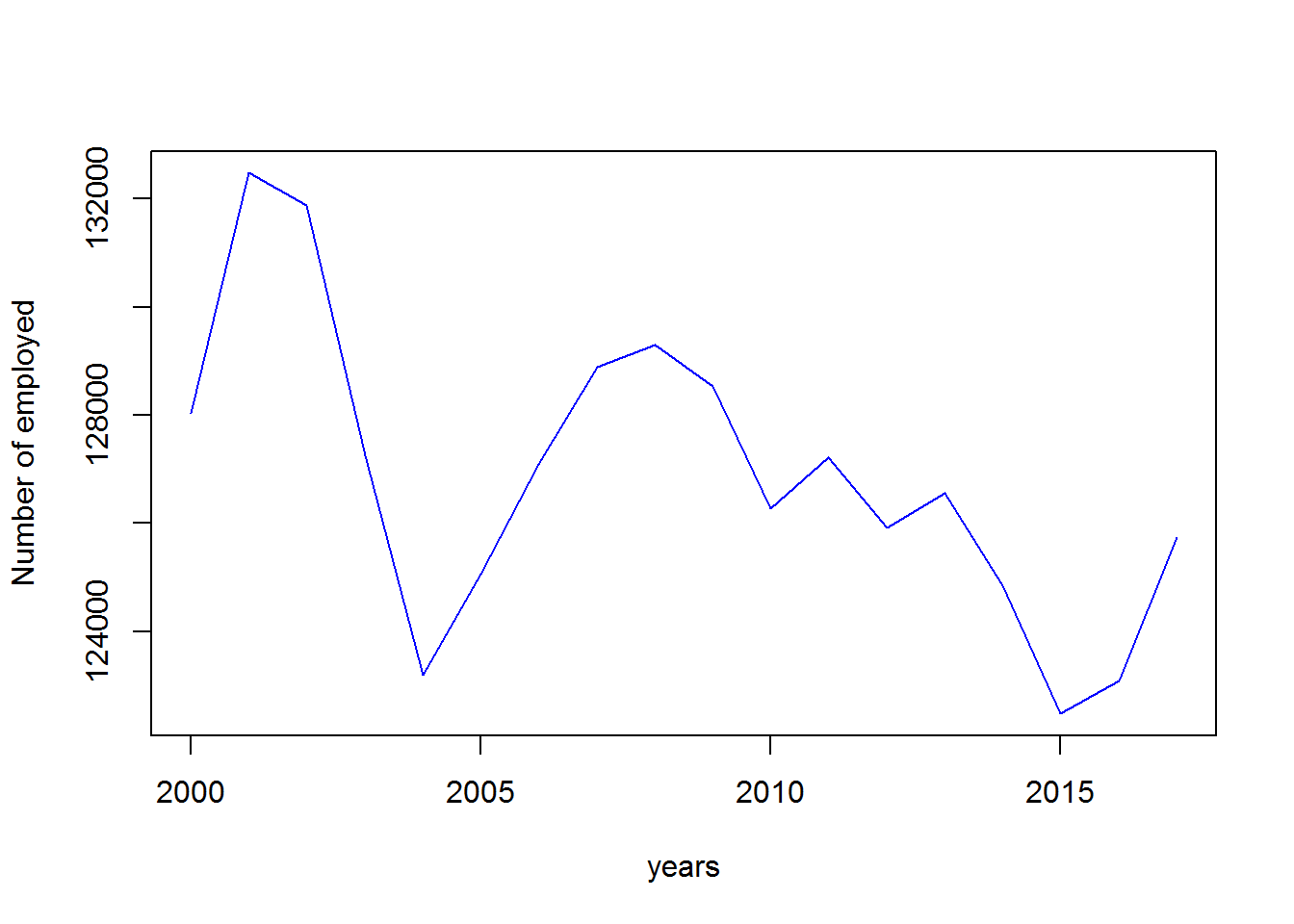### Time Series using ts() function The ts() function will convert a numeric vector into an R time series object. We have to specify the start date, the end and the frequency • Start date: 2000 • End date: 2017 • Frequency: 1 if annual, 4 if quartly and 12 if monthly myts<-ts(TSQuebec$Employed, start=c(2000, 1), frequency=1)
plot(myts,las=2)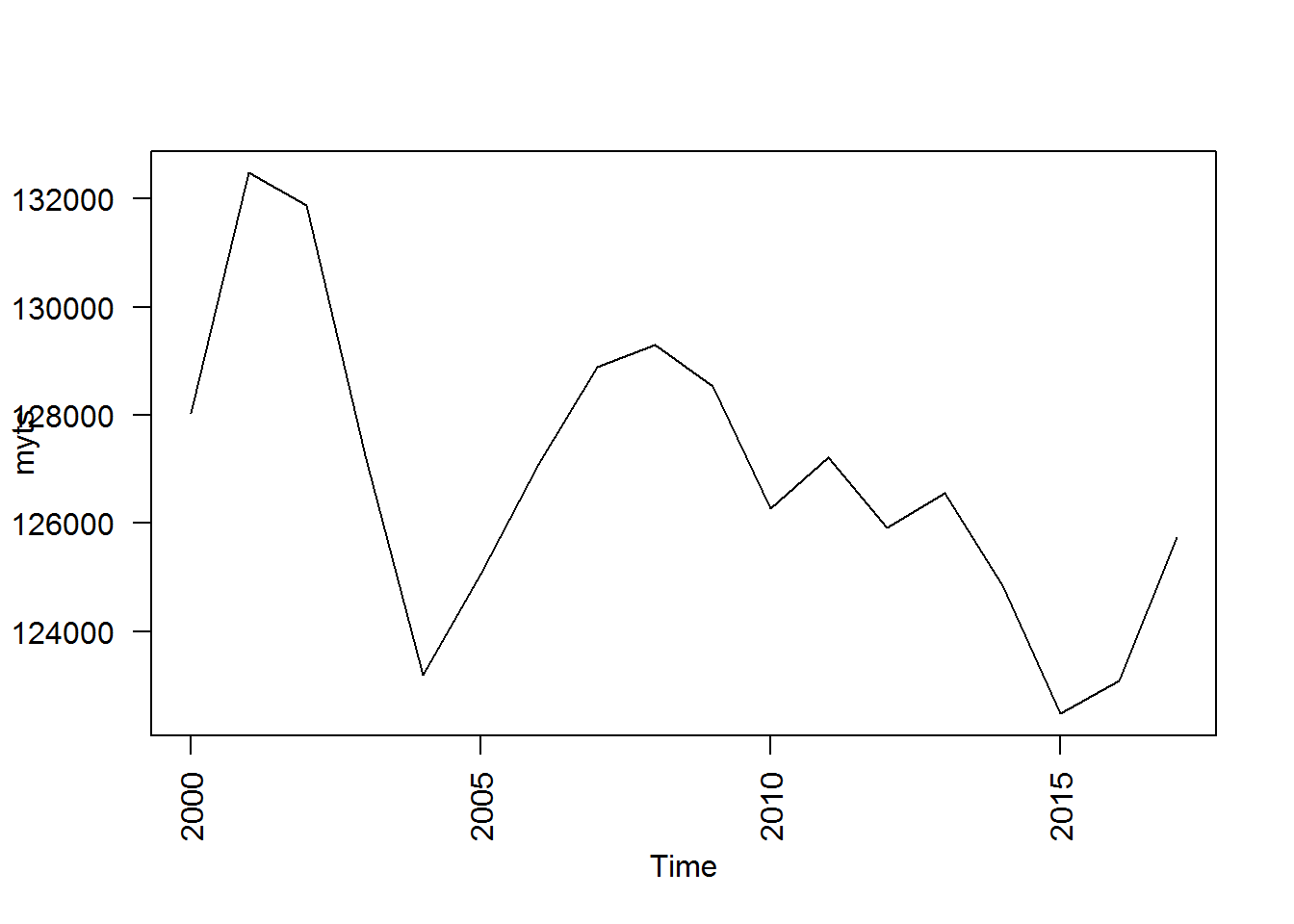Plot several time series on a common plot using ts.plot().

-First: Create the time series.

mytsON=ts(subset(TSCanada,prov=='Ontario')$hrlyearn,start=c(2000,1),frequency=1) mytsQC=ts(subset(TSCanada,prov=='Quebec')$hrlyearn,start=c(2000, 1),frequency=1)
myts=cbind(mytsQC,mytsON,mytsBC)
myts
## Time Series:
## Start = 2000
## End = 2017
## Frequency = 1
##      mytsQC mytsON mytsBC
## 2000     30     49     56
## 2001     35     59     61
## 2002     40     65     69
## 2003     46     72     73
## 2004     55     82     77
## 2005     62     89     78
## 2006     68     97     88
## 2007     79    109     98
## 2008     90    120    116
## 2009    102    130    127
## 2010    105    136    133
## 2011    113    142    138
## 2012    121    147    146
## 2013    129    154    150
## 2014    137    159    156
## 2015    145    166    164
## 2016    152    170    167
## 2017    161    172    169
• Second: Plot the evolution of average wage in Quebec, British Columbia and Ontario.
require(graphics)
ts.plot(myts,gpars=list(ylab="Hourly wagee", lty=c(1:3)))
legend("topleft",legend =c("Ontario","Quebec","British Columbia"), lty=c(1:3))